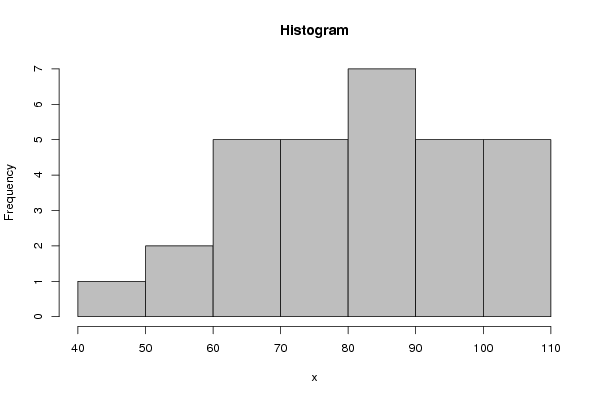## Free Statistics

of Irreproducible Research!

Author's title
Author*The author of this computation has been verified*
R Software Modulerwasp_histogram.wasp
Title produced by softwareHistogram
Date of computationWed, 22 Dec 2010 14:20:36 +0000
Cite this page as followsStatistical Computations at FreeStatistics.org, Office for Research Development and Education, URL https://freestatistics.org/blog/index.php?v=date/2010/Dec/22/t1293027560577n1760gfwhzn3.htm/, Retrieved Sat, 30 Sep 2023 13:14:45 +0000
Statistical Computations at FreeStatistics.org, Office for Research Development and Education, URL https://freestatistics.org/blog/index.php?pk=114241, Retrieved Sat, 30 Sep 2023 13:14:45 +0000
QR Codes:Original text written by user:
IsPrivate?No (this computation is public)
User-defined keywords
Estimated Impact137
Family? (F = Feedback message, R = changed R code, M = changed R Module, P = changed Parameters, D = changed Data)
-     [Univariate Data Series] [Arabica Price in ...] [2008-01-05 23:43:18] [74be16979710d4c4e7c6647856088456]
- RMPD  [Histogram] [histogram inflatie] [2010-12-22 13:21:55] [96348ef82925ade81ab3c243141d80f1]
-    D      [Histogram] [Historgram Inflatie] [2010-12-22 14:20:36] [694c30abd2a3b2ee5cb46fc74cb5bfb9] [Current]
-    D        [Histogram] [Histogram BBP] [2010-12-22 14:24:29] [30b3e197115d238a51c18bcedc33a6a5]
- RMPD        [Bootstrap Plot - Central Tendency] [blocked bootstrap] [2010-12-22 14:35:50] [30b3e197115d238a51c18bcedc33a6a5]
-    D          [Bootstrap Plot - Central Tendency] [notched boxplots ...] [2010-12-22 17:51:05] [96348ef82925ade81ab3c243141d80f1]
- RMPD        [Bootstrap Plot - Central Tendency] [blocked bootstrap...] [2010-12-22 14:40:22] [30b3e197115d238a51c18bcedc33a6a5]
Feedback Forum

Post a new message
Dataseries X:
47.91
51.56
56.06
60.36
64.19
67.31
68.18
69.24
70.05
72.22
74.72
77.08
78.81
80.78
82.71
83.76
85.26
86.53
87.32
88.31
90.67
92.88
94.33
95.75
97.53
100
102.33
104.19
108.87
108.86


 Summary of computational transaction Raw Input view raw input (R code) Raw Output view raw output of R engine Computing time 32 seconds R Server 'George Udny Yule' @ 72.249.76.132

\begin{tabular}{lllllllll}
\hline
Summary of computational transaction \tabularnewline
Raw Input & view raw input (R code)  \tabularnewline
Raw Output & view raw output of R engine  \tabularnewline
Computing time & 32 seconds \tabularnewline
R Server & 'George Udny Yule' @ 72.249.76.132 \tabularnewline
\hline
\end{tabular}
%Source: https://freestatistics.org/blog/index.php?pk=114241&T=0

[TABLE]
[ROW][C]Summary of computational transaction[/C][/ROW]
[ROW][C]Raw Input[/C][C]view raw input (R code) [/C][/ROW]
[ROW][C]Raw Output[/C][C]view raw output of R engine [/C][/ROW]
[ROW][C]Computing time[/C][C]32 seconds[/C][/ROW]
[ROW][C]R Server[/C][C]'George Udny Yule' @ 72.249.76.132[/C][/ROW]
[/TABLE]
Source: https://freestatistics.org/blog/index.php?pk=114241&T=0

Globally Unique Identifier (entire table): ba.freestatistics.org/blog/index.php?pk=114241&T=0

As an alternative you can also use a QR Code:

The GUIDs for individual cells are displayed in the table below:

 Summary of computational transaction Raw Input view raw input (R code) Raw Output view raw output of R engine Computing time 32 seconds R Server 'George Udny Yule' @ 72.249.76.132

 Frequency Table (Histogram) Bins Midpoint Abs. Frequency Rel. Frequency Cumul. Rel. Freq. Density [40,50[ 45 1 0.033333 0.033333 0.003333 [50,60[ 55 2 0.066667 0.1 0.006667 [60,70[ 65 5 0.166667 0.266667 0.016667 [70,80[ 75 5 0.166667 0.433333 0.016667 [80,90[ 85 7 0.233333 0.666667 0.023333 [90,100[ 95 5 0.166667 0.833333 0.016667 [100,110] 105 5 0.166667 1 0.016667

\begin{tabular}{lllllllll}
\hline
Frequency Table (Histogram) \tabularnewline
Bins & Midpoint & Abs. Frequency & Rel. Frequency & Cumul. Rel. Freq. & Density \tabularnewline
[40,50[ & 45 & 1 & 0.033333 & 0.033333 & 0.003333 \tabularnewline
[50,60[ & 55 & 2 & 0.066667 & 0.1 & 0.006667 \tabularnewline
[60,70[ & 65 & 5 & 0.166667 & 0.266667 & 0.016667 \tabularnewline
[70,80[ & 75 & 5 & 0.166667 & 0.433333 & 0.016667 \tabularnewline
[80,90[ & 85 & 7 & 0.233333 & 0.666667 & 0.023333 \tabularnewline
[90,100[ & 95 & 5 & 0.166667 & 0.833333 & 0.016667 \tabularnewline
[100,110] & 105 & 5 & 0.166667 & 1 & 0.016667 \tabularnewline
\hline
\end{tabular}
%Source: https://freestatistics.org/blog/index.php?pk=114241&T=1

[TABLE]
[ROW][C]Frequency Table (Histogram)[/C][/ROW]
[ROW][C]Bins[/C][C]Midpoint[/C][C]Abs. Frequency[/C][C]Rel. Frequency[/C][C]Cumul. Rel. Freq.[/C][C]Density[/C][/ROW]
[ROW][C][40,50[[/C][C]45[/C][C]1[/C][C]0.033333[/C][C]0.033333[/C][C]0.003333[/C][/ROW]
[ROW][C][50,60[[/C][C]55[/C][C]2[/C][C]0.066667[/C][C]0.1[/C][C]0.006667[/C][/ROW]
[ROW][C][60,70[[/C][C]65[/C][C]5[/C][C]0.166667[/C][C]0.266667[/C][C]0.016667[/C][/ROW]
[ROW][C][70,80[[/C][C]75[/C][C]5[/C][C]0.166667[/C][C]0.433333[/C][C]0.016667[/C][/ROW]
[ROW][C][80,90[[/C][C]85[/C][C]7[/C][C]0.233333[/C][C]0.666667[/C][C]0.023333[/C][/ROW]
[ROW][C][90,100[[/C][C]95[/C][C]5[/C][C]0.166667[/C][C]0.833333[/C][C]0.016667[/C][/ROW]
[ROW][C][100,110][/C][C]105[/C][C]5[/C][C]0.166667[/C][C]1[/C][C]0.016667[/C][/ROW]
[/TABLE]
Source: https://freestatistics.org/blog/index.php?pk=114241&T=1

Globally Unique Identifier (entire table): ba.freestatistics.org/blog/index.php?pk=114241&T=1

As an alternative you can also use a QR Code:

The GUIDs for individual cells are displayed in the table below:

 Frequency Table (Histogram) Bins Midpoint Abs. Frequency Rel. Frequency Cumul. Rel. Freq. Density [40,50[ 45 1 0.033333 0.033333 0.003333 [50,60[ 55 2 0.066667 0.1 0.006667 [60,70[ 65 5 0.166667 0.266667 0.016667 [70,80[ 75 5 0.166667 0.433333 0.016667 [80,90[ 85 7 0.233333 0.666667 0.023333 [90,100[ 95 5 0.166667 0.833333 0.016667 [100,110] 105 5 0.166667 1 0.016667PNG link Postscript link PDF link

Parameters (Session):
par2 = grey ; par3 = FALSE ; par4 = Unknown ;
Parameters (R input):
par1 = ; par2 = grey ; par3 = FALSE ; par4 = Unknown ;
R code (references can be found in the software module):
par1 <- as.numeric(par1)if (par3 == 'TRUE') par3 <- TRUEif (par3 == 'FALSE') par3 <- FALSEif (par4 == 'Unknown') par1 <- as.numeric(par1)if (par4 == 'Interval/Ratio') par1 <- as.numeric(par1)if (par4 == '3-point Likert') par1 <- c(1:3 - 0.5, 3.5)if (par4 == '4-point Likert') par1 <- c(1:4 - 0.5, 4.5)if (par4 == '5-point Likert') par1 <- c(1:5 - 0.5, 5.5)if (par4 == '6-point Likert') par1 <- c(1:6 - 0.5, 6.5)if (par4 == '7-point Likert') par1 <- c(1:7 - 0.5, 7.5)if (par4 == '8-point Likert') par1 <- c(1:8 - 0.5, 8.5)if (par4 == '9-point Likert') par1 <- c(1:9 - 0.5, 9.5)if (par4 == '10-point Likert') par1 <- c(1:10 - 0.5, 10.5)bitmap(file='test1.png')if(is.numeric(x)) {if (is.na(par1)) {myhist<-hist(x,col=par2,main=main,xlab=xlab,right=par3)} else {if (par1 < 0) par1 <- 3if (par1 > 50) par1 <- 50myhist<-hist(x,breaks=par1,col=par2,main=main,xlab=xlab,right=par3)}} else {plot(mytab <- table(x),col=par2,main='Frequency Plot',xlab=xlab,ylab='Absolute Frequency')}dev.off()if(is.numeric(x)) {myhistn <- length(x)load(file='createtable')a<-table.start()a<-table.row.start(a)a<-table.element(a,hyperlink('histogram.htm','Frequency Table (Histogram)',''),6,TRUE)a<-table.row.end(a)a<-table.row.start(a)a<-table.element(a,'Bins',header=TRUE)a<-table.element(a,'Midpoint',header=TRUE)a<-table.element(a,'Abs. Frequency',header=TRUE)a<-table.element(a,'Rel. Frequency',header=TRUE)a<-table.element(a,'Cumul. Rel. Freq.',header=TRUE)a<-table.element(a,'Density',header=TRUE)a<-table.row.end(a)crf <- 0if (par3 == FALSE) mybracket <- '[' else mybracket <- ']'mynumrows <- (length(myhist$breaks)-1)for (i in 1:mynumrows) {a<-table.row.start(a)if (i == 1)dum <- paste('[',myhist$breaks[i],sep='')elsedum <- paste(mybracket,myhist$breaks[i],sep='')dum <- paste(dum,myhist$breaks[i+1],sep=',')if (i==mynumrows)dum <- paste(dum,']',sep='')elsedum <- paste(dum,mybracket,sep='')a<-table.element(a,dum,header=TRUE)a<-table.element(a,myhist$mids[i])a<-table.element(a,myhist$counts[i])rf <- myhist$counts[i]/ncrf <- crf + rfa<-table.element(a,round(rf,6))a<-table.element(a,round(crf,6))a<-table.element(a,round(myhist$density[i],6))a<-table.row.end(a)}a<-table.end(a)table.save(a,file='mytable.tab')} else {mytabreltab <- mytab / sum(mytab)n <- length(mytab)load(file='createtable')a<-table.start()a<-table.row.start(a)a<-table.element(a,'Frequency Table (Categorical Data)',3,TRUE)a<-table.row.end(a)a<-table.row.start(a)a<-table.element(a,'Category',header=TRUE)a<-table.element(a,'Abs. Frequency',header=TRUE)a<-table.element(a,'Rel. Frequency',header=TRUE)a<-table.row.end(a)for (i in 1:n) {a<-table.row.start(a)a<-table.element(a,labels(mytab)\$x[i],header=TRUE)a<-table.element(a,mytab[i])a<-table.element(a,round(reltab[i],4))a<-table.row.end(a)}a<-table.end(a)table.save(a,file='mytable1.tab')}